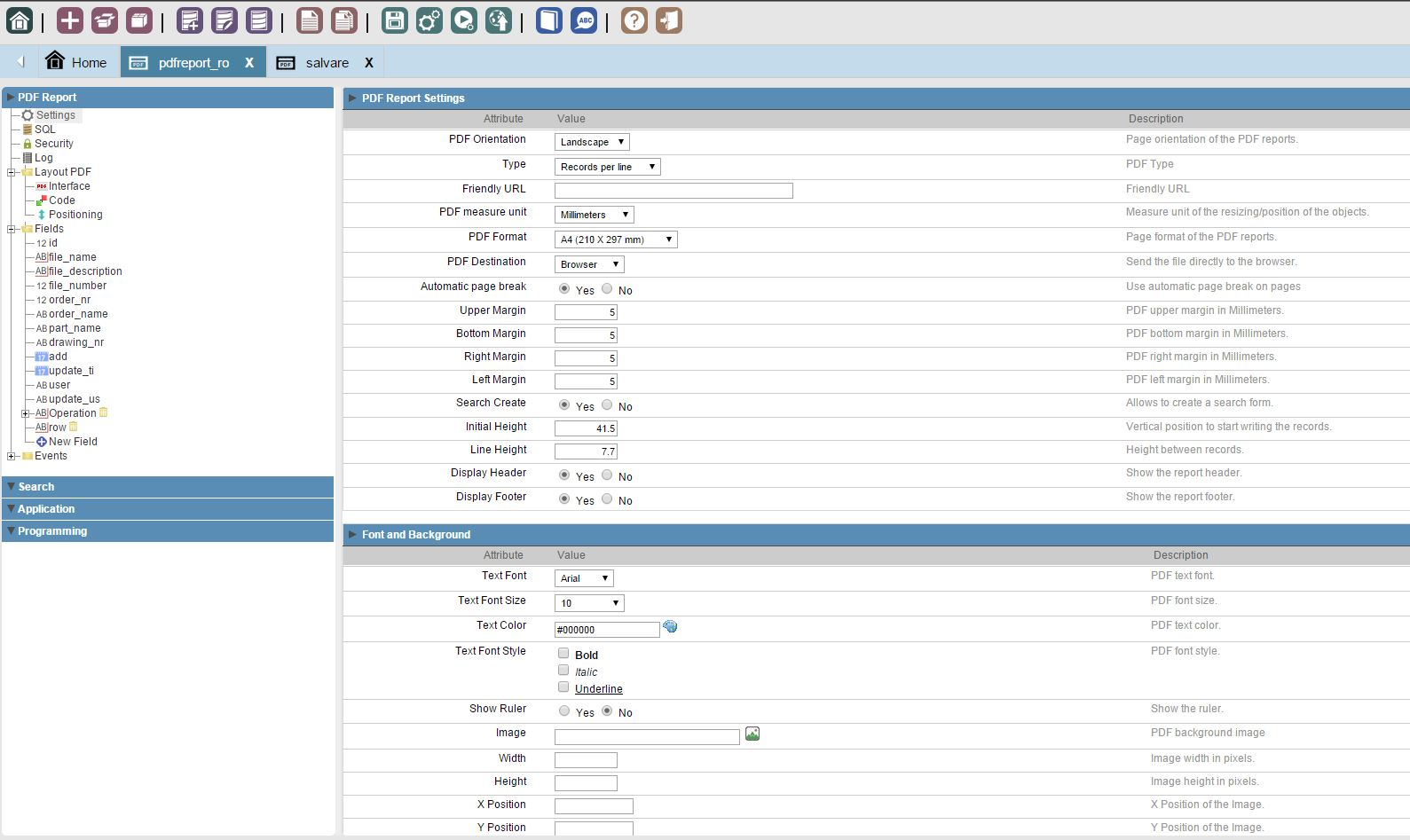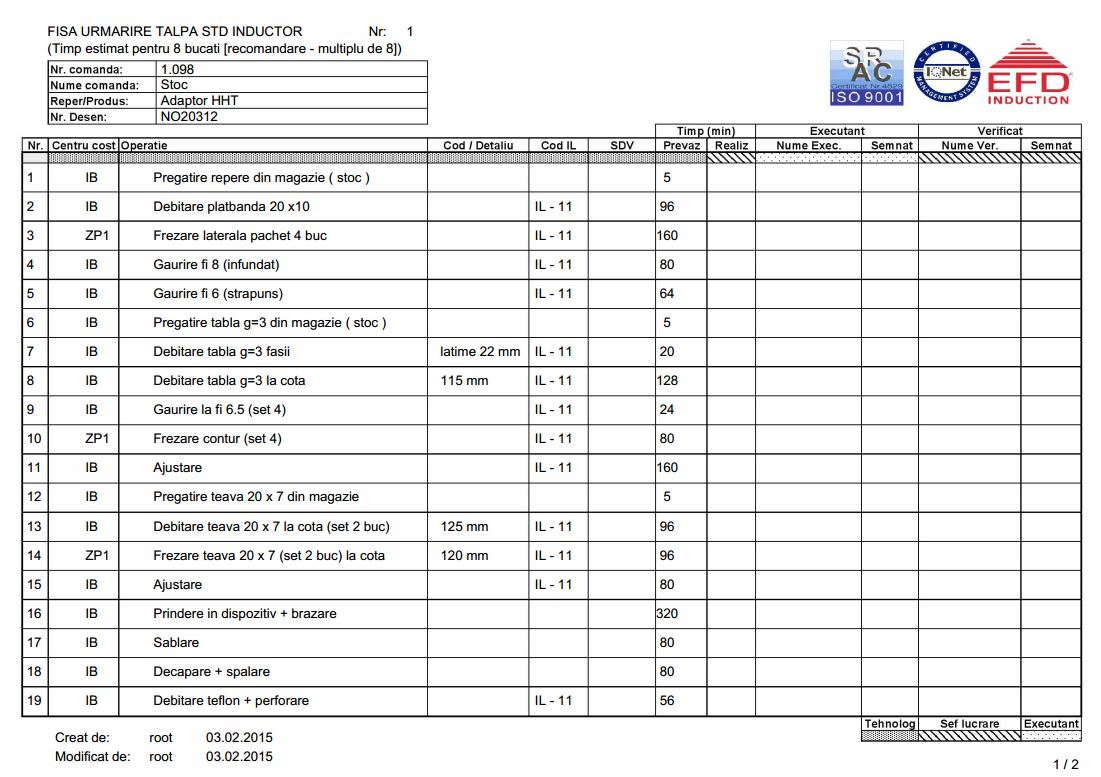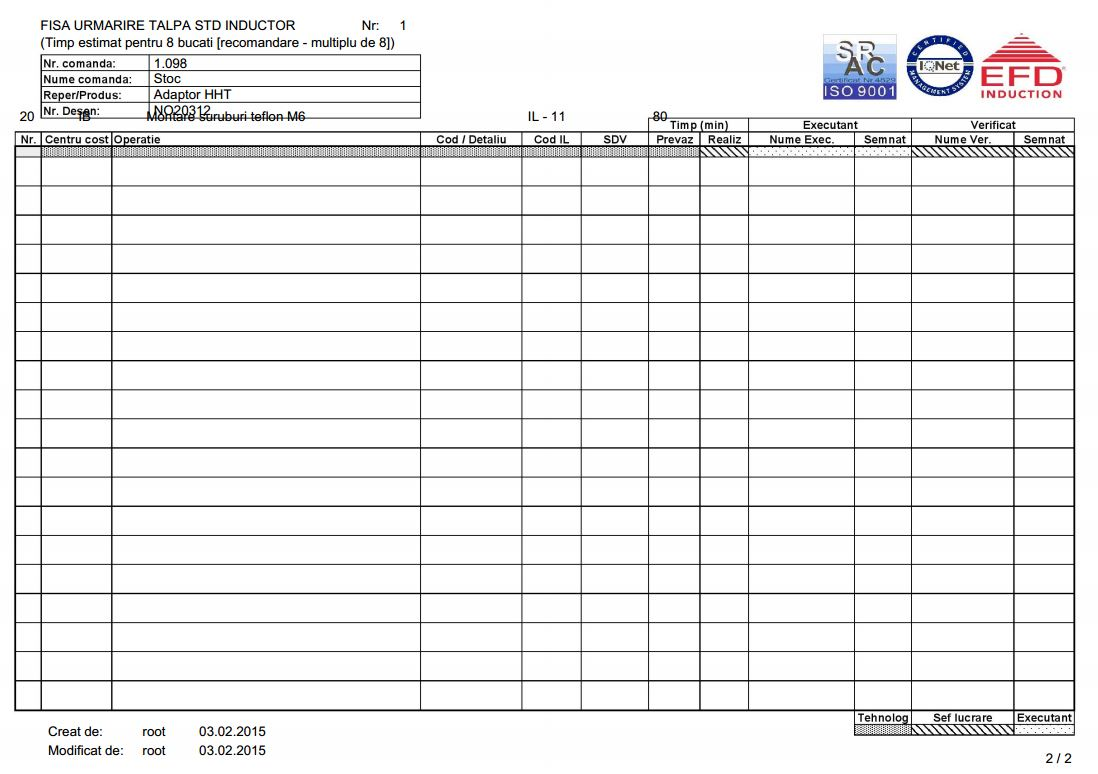# pdf report - incorrect second page display

Hello everyone,

I’m trying to create a pdf report that has an image as background, an subselect and also a header and a footer.

The proablem is that when i go to the second page scriptcase forget about my header and footer and write the information from subselect over them.
#####################
This is my version of scripcase: #
#
Version 8.00.0030 #
Types pe_mysql_bronze #
#####################

This is my cod:
/------------------ Page 1 -----------------/

``````	sc_pdf_print_mult(\$cell_row, 3.5277777777778);
sc_pdf_sub_sel_init();
sc_pdf_set_y(43);
foreach ({Operation} as \$NM_ind =&gt; \$Dados)
{
sc_pdf_print_sub_sel(\$cell_Operation_cost_center[\$NM_ind]);
sc_pdf_print_sub_sel(\$cell_Operation_operation[\$NM_ind]);
sc_pdf_print_sub_sel(\$cell_Operation_parts_cod[\$NM_ind]);
sc_pdf_print_sub_sel(\$cell_Operation_wi_cod[\$NM_ind]);
sc_pdf_print_sub_sel(\$cell_Operation_sdv[\$NM_ind]);
sc_pdf_print_sub_sel(\$cell_Operation_stipulated[\$NM_ind]);
sc_pdf_print_sub_sel(\$cell_Operation_worker_name[\$NM_ind]);
sc_pdf_print_sub_sel(\$cell_Operation_checker[\$NM_ind]);
/*sc_pdf_ln(7.17);*/
sc_pdf_sub_sel_end();
sc_pdf_set_auto_page_break('auto',20);
\$i = sc_pdf_get_y(); /*Returns the ordinate of the current position and asing to \$i*/

if (\$i &gt; 249)
{
\$i = 43; /* this its the magic line. asign new valor to the ordinate position*/
}

}

/*-------------------------------------------*/
``````

Does anyone have any ideea where i got mistaken?

Attaced are some details as: fields - operation, settings, layout - interface and the result of the pdf report

P.S. 1) Does anyone knows where i could find the commands and macros from scriptcase explained better then the scriptcase manual ?;
2) Does anyone find on the internet a place that has a lot more examples on how to use commands and macros from scriptcase ?.

Thank you all for your time and help,
Dan

pdf report with subselect, image background, image attaced, header and footer - incorrect second page display;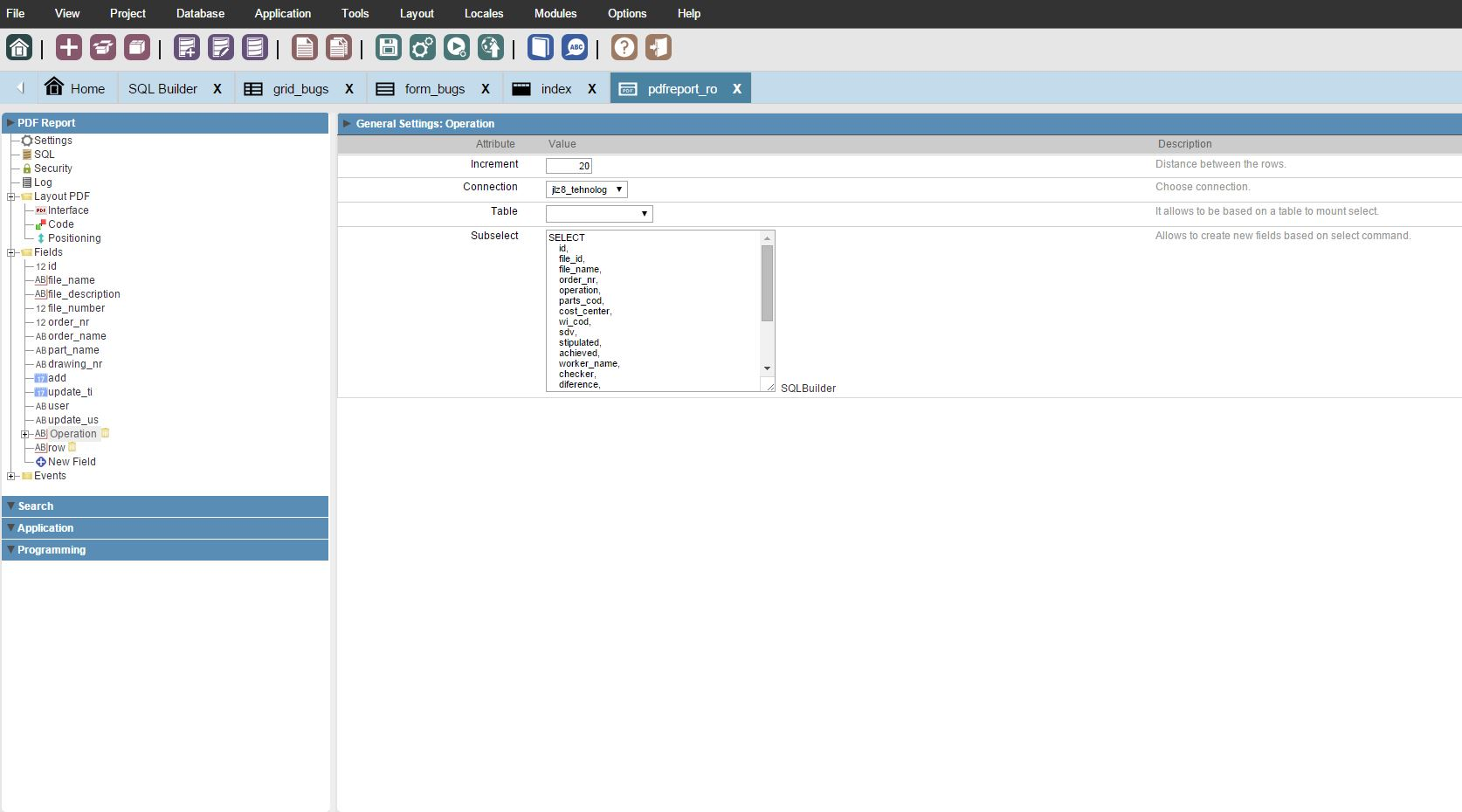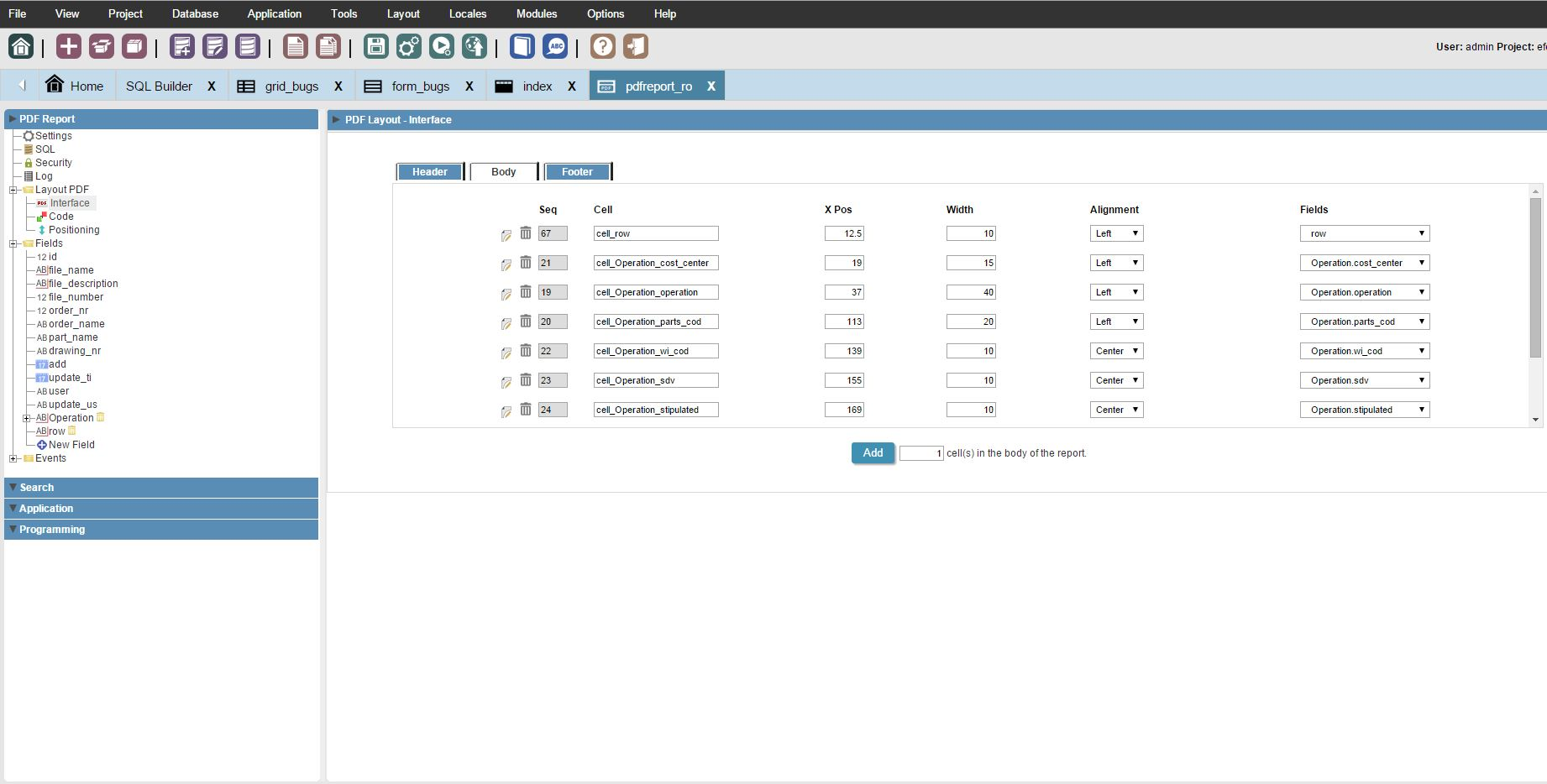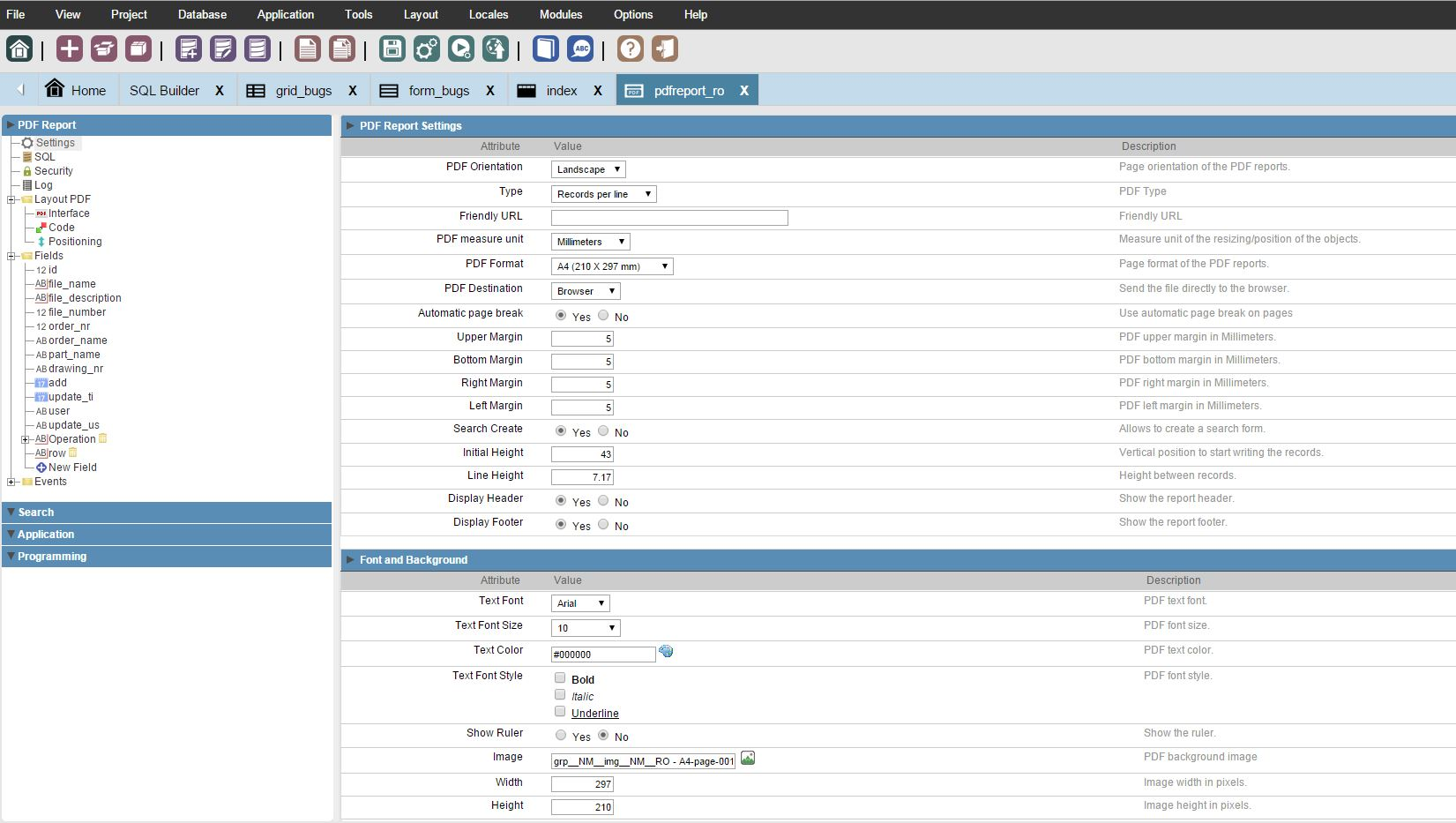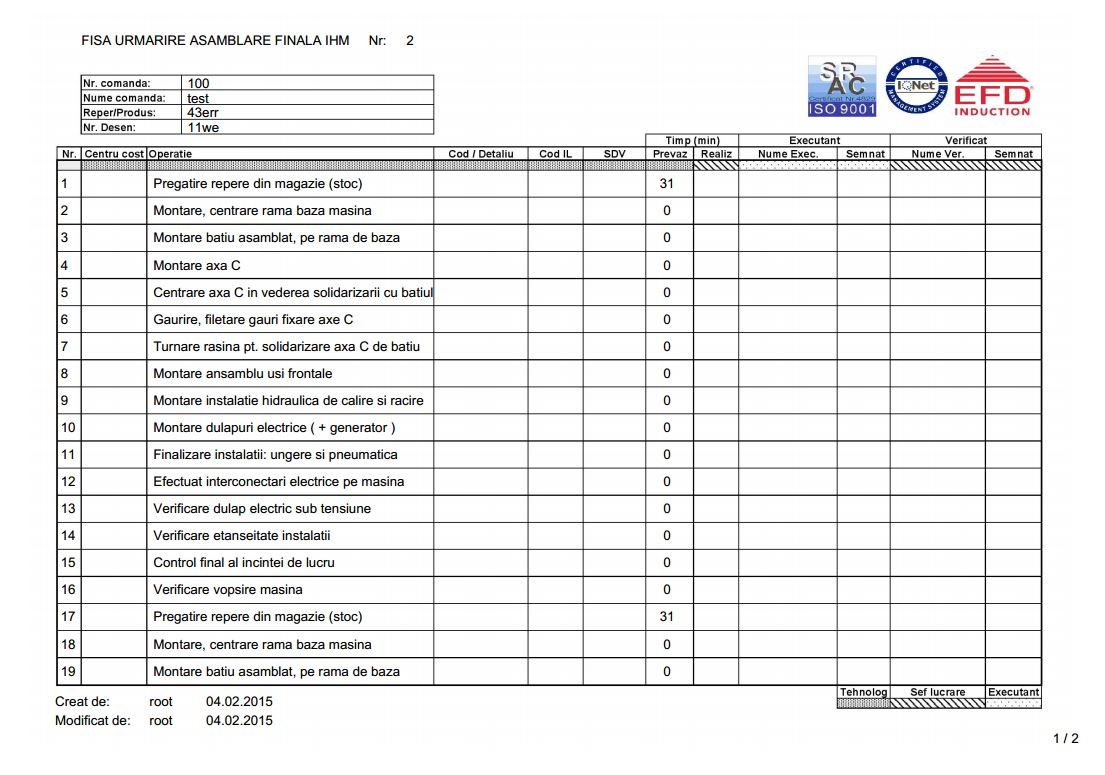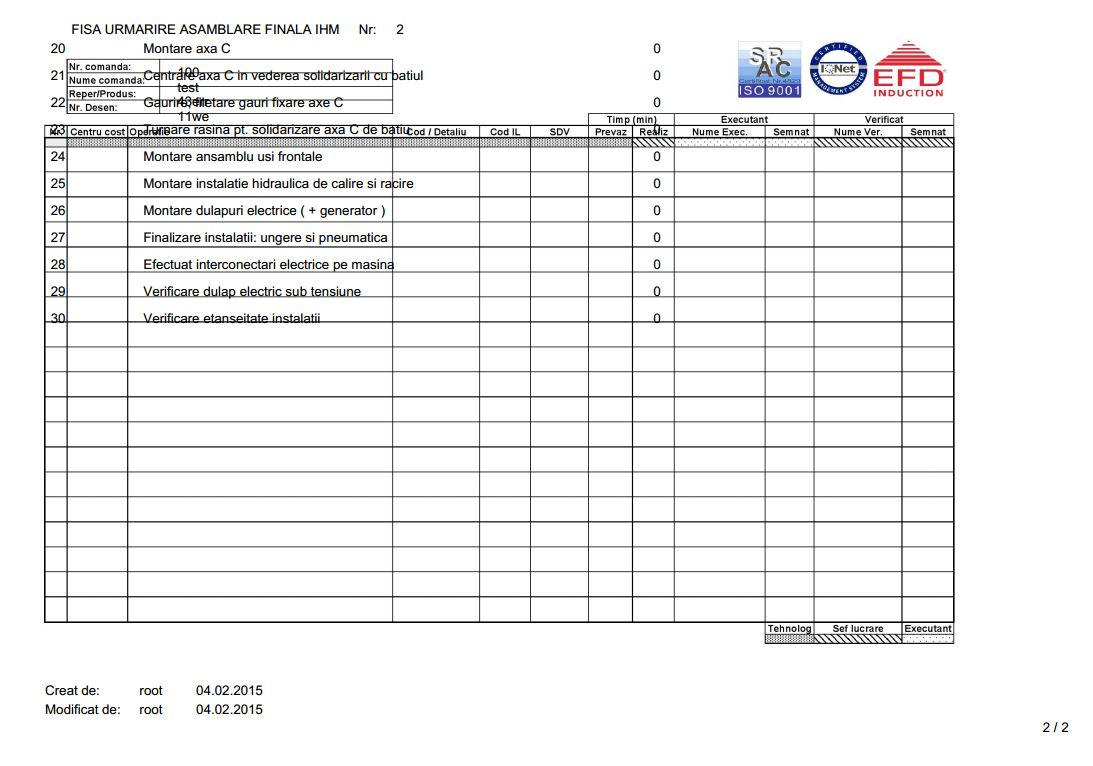This is a bug in SC … When you set AutoPageBreak to yes SC forget restore this option.

Try the following at Layout PDF / Code / Header before the autogenerated code:

// Quelle: http://www.tcpdf.org/examples/example_051.phps
// get the current page break margin
\$bMargin = \$this->getBreakMargin();
// get current auto-page-break mode
\$auto_page_break = \$this->AutoPageBreak;
// disable auto-page-break
\$this->SetAutoPageBreak(false, 0);
// set background image
\$img_file = ‘…/_lib/img/sys__NM__zeitnachweis.png’;
\$this->Image(\$img_file, 0, 0, 210, 297, ‘’, ‘’, ‘’, false, 300, ‘’, false, false, 0);
// restore auto-page-break status
\$this->SetAutoPageBreak(\$auto_page_break, \$bMargin);
// set the starting point for the page content
\$this->setPageMark();

Hello,

Thank you for your quick unser but i didn’t succided to solve the proablem…i thing i didn’t understand well how to use that library. (here is my updated cod - can you please correct it where i got rong).

``````      /*------------------ Page 1 -----------------*/

sc_pdf_print_mult(\$cell_row, 3.5277777777778);
sc_pdf_sub_sel_init();
sc_pdf_set_y(43);
foreach ({Operation} as \$NM_ind =&gt; \$Dados)
{
sc_pdf_print_sub_sel(\$cell_Operation_cost_center[\$NM_ind]);
sc_pdf_print_sub_sel(\$cell_Operation_operation[\$NM_ind]);
sc_pdf_print_sub_sel(\$cell_Operation_parts_cod[\$NM_ind]);
sc_pdf_print_sub_sel(\$cell_Operation_wi_cod[\$NM_ind]);
sc_pdf_print_sub_sel(\$cell_Operation_sdv[\$NM_ind]);
sc_pdf_print_sub_sel(\$cell_Operation_stipulated[\$NM_ind]);
sc_pdf_print_sub_sel(\$cell_Operation_worker_name[\$NM_ind]);
sc_pdf_print_sub_sel(\$cell_Operation_checker[\$NM_ind]);
/*sc_pdf_ln(7.17);*/
sc_pdf_sub_sel_end();
sc_pdf_set_auto_page_break('auto',20);
\$i = sc_pdf_get_y(); /*Returns the ordinate of the current position and asing to \$i*/

if (\$i &gt; 249)
{
// get the current page break margin
\$bMargin = \$this-&gt;getBreakMargin();
// get current auto-page-break mode
\$auto_page_break = \$this-&gt;AutoPageBreak;
// disable auto-page-break
\$this-&gt;SetAutoPageBreak(false, 0);
// restore auto-page-break status
\$this-&gt;SetAutoPageBreak(\$auto_page_break, \$bMargin);
// set the starting point for the page content
\$this-&gt;setPageMark();

\$i = 43; /* this its the magic line. asign new valor to the ordinate position*/
}

}

/*-------------------------------------------*/
``````

Dan

If have written, that you should insert my code in the header section before the generated code. You have insert that in the body section …

If i put it in the header i recive this error: - Parse error: syntax error, unexpected ‘{’ in /opt/NetMake/v8/wwwroot/scriptcase/app/efd_tehnolog/pdfreport_ro/pdfreport_ro_grid.class.php on line 804

``````      /*------------------ Page 1 -----------------*/
``````

{
// get the current page break margin
\$bMargin = \$this->getBreakMargin();
// get current auto-page-break mode
\$auto_page_break = \$this->AutoPageBreak;
// disable auto-page-break
\$this->SetAutoPageBreak(false, 0);
// restore auto-page-break status
\$this->SetAutoPageBreak(\$auto_page_break, \$bMargin);
// set the starting point for the page content
\$this->setPageMark();
}

``````	sc_pdf_print_mult(\$cell_row, 3.5277777777778);
sc_pdf_sub_sel_init();
sc_pdf_set_y(43);
foreach ({Operation} as \$NM_ind =&gt; \$Dados)
{
sc_pdf_print_sub_sel(\$cell_Operation_cost_center[\$NM_ind]);
sc_pdf_print_sub_sel(\$cell_Operation_operation[\$NM_ind]);
sc_pdf_print_sub_sel(\$cell_Operation_parts_cod[\$NM_ind]);
sc_pdf_print_sub_sel(\$cell_Operation_wi_cod[\$NM_ind]);
sc_pdf_print_sub_sel(\$cell_Operation_sdv[\$NM_ind]);
sc_pdf_print_sub_sel(\$cell_Operation_stipulated[\$NM_ind]);
sc_pdf_print_sub_sel(\$cell_Operation_worker_name[\$NM_ind]);
sc_pdf_print_sub_sel(\$cell_Operation_checker[\$NM_ind]);
/*sc_pdf_ln(7.17);*/
sc_pdf_sub_sel_end();
sc_pdf_set_auto_page_break('auto',20);
\$i = sc_pdf_get_y(); /*Returns the ordinate of the current position and asing to \$i*/

if (\$i &gt; 249)
{
\$i = 43; /* this its the magic line. asign new valor to the ordinate position*/
}

}

/*-------------------------------------------*/``````

I wrote header, i wrote not in top of the body code …

And, remove your own code from the body section. This is not functional …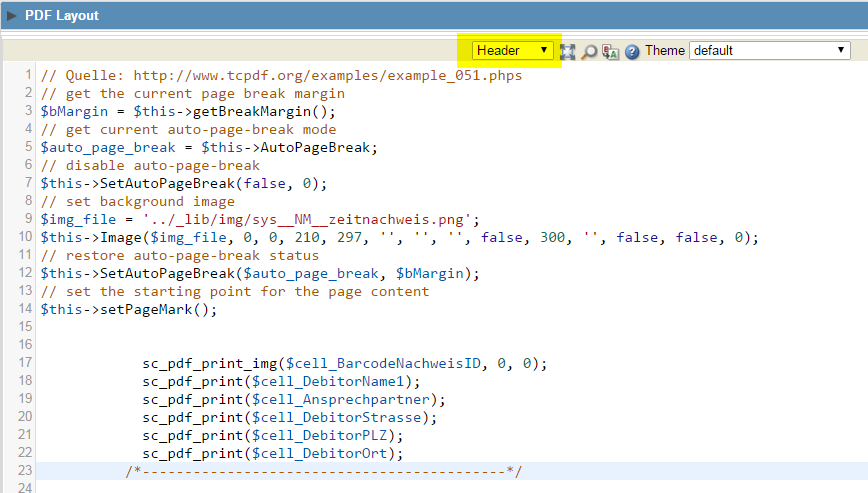same resultI tryed this code (your code):

###################################################################
// Quelle: http://www.tcpdf.org/examples/example_051.phps
// get the current page break margin
\$bMargin = \$this->getBreakMargin();
// get current auto-page-break mode
\$auto_page_break = \$this->AutoPageBreak;
// disable auto-page-break
\$this->SetAutoPageBreak(false, 0);
// restore auto-page-break status
\$this->SetAutoPageBreak(\$auto_page_break, \$bMargin);
// set the starting point for the page content
\$this->setPageMark();
###################################################################

I inserted in the header first, after that in layout, after that in footer and after that in header and layout and the result was the same…attached are photos from my tries.

Do you have any suggestion?

Dan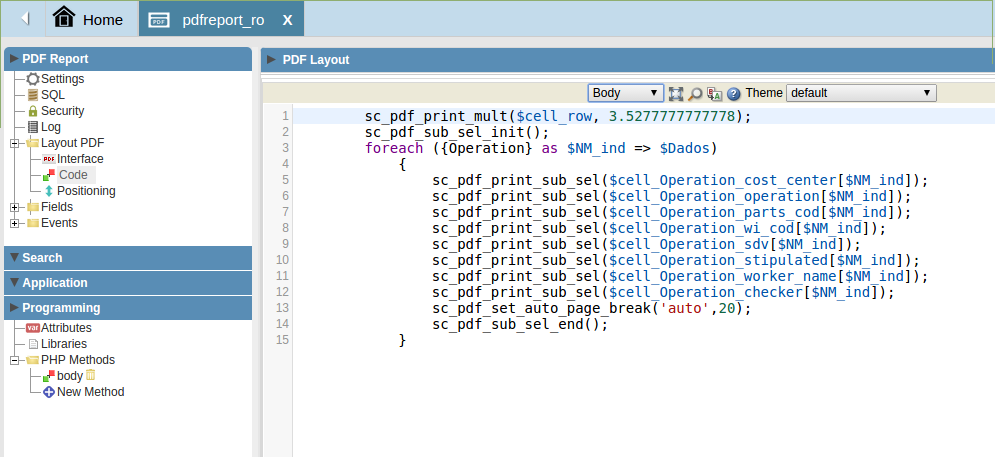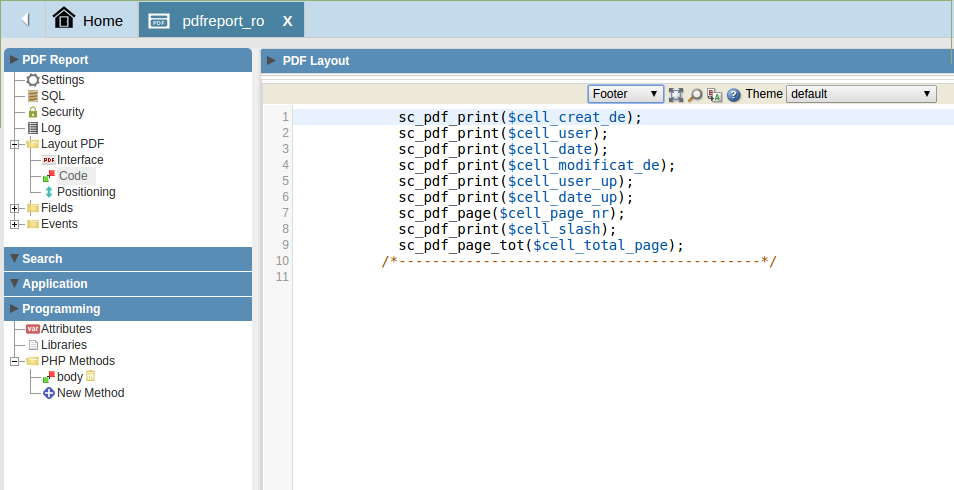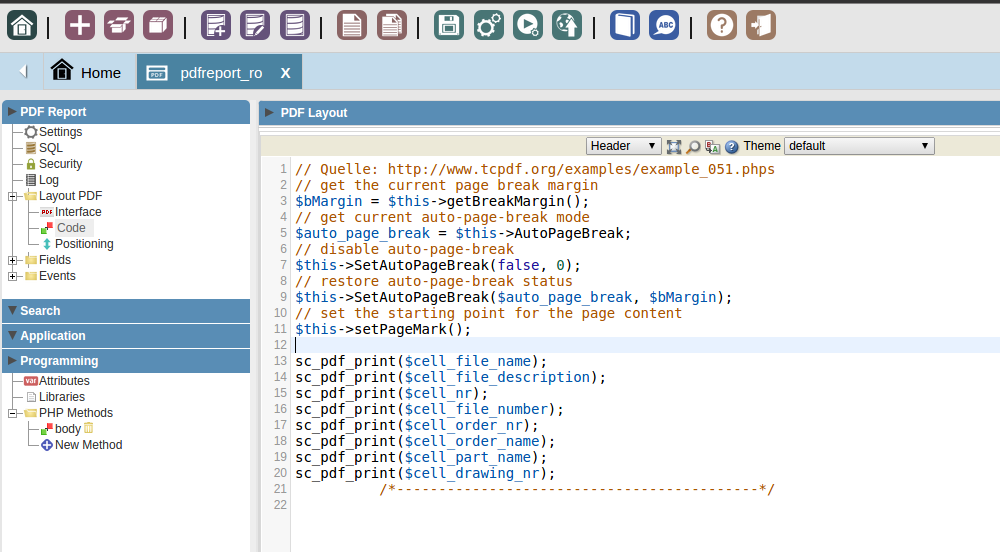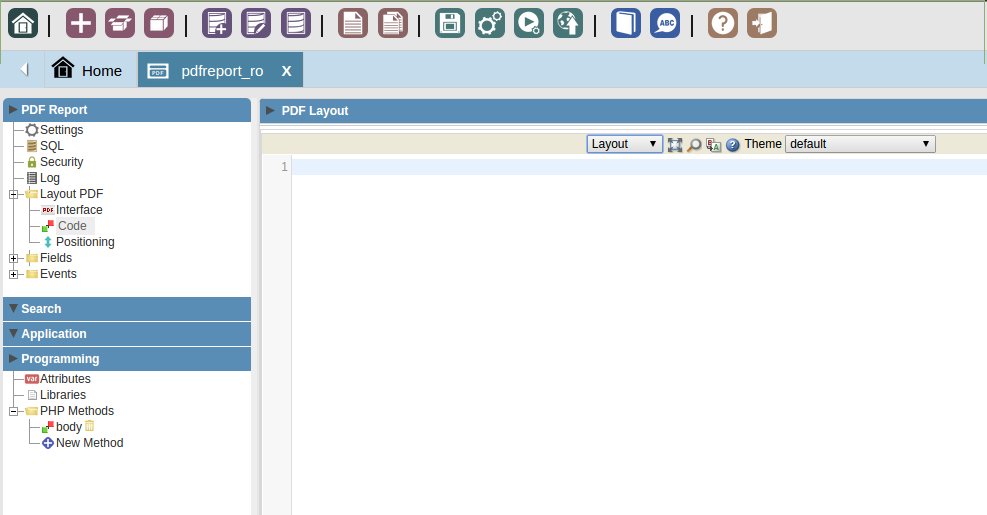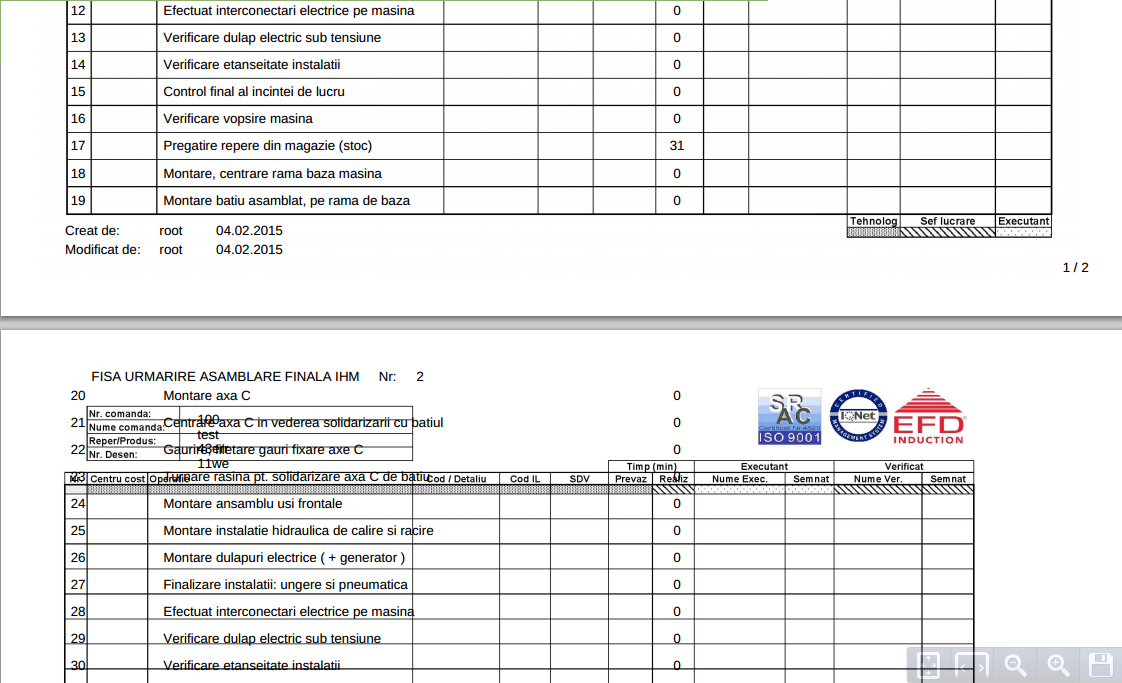Yes, but I wonder if it makes sense want to help you more … You’ve got a problem with your background image and the report header. Why your are shortened my code example and clears the area with the image? So it makes no sense … Insert your image name and path in my code example and clear the image details in the app settings.sorry for that, i thought i don’t nead that.

After i study a little bit the documentation of tcpdf and made the next modifications - i advanced a little bit - the text almost jump over the header…but still not going where it needs to be.

Layout:
##############################################################################################
\$pdf = new TCPDF(PDF_PAGE_ORIENTATION, PDF_UNIT, PDF_PAGE_FORMAT, true, ‘UTF-8’, false);

// set document information
// \$pdf->SetCreator(PDF_CREATOR);
// \$pdf->SetAuthor(‘Ronyan Alves’);
// \$pdf->SetTitle(‘Scriptcase TCPDF’);
// \$pdf->SetSubject(‘TCPDF Webnar’);

\$pdf->setPrintFooter(false);

// set default monospaced font
\$pdf->SetDefaultMonospacedFont(PDF_FONT_MONOSPACED);

// set margins
\$pdf->SetMargins(PDF_MARGIN_LEFT, PDF_MARGIN_TOP, PDF_MARGIN_RIGHT);
\$pdf->SetFooterMargin(PDF_MARGIN_FOOTER);

// set auto page breaks
\$pdf->SetAutoPageBreak(TRUE, PDF_MARGIN_BOTTOM);

// set image scale factor
\$pdf->setImageScale(PDF_IMAGE_SCALE_RATIO);

// set some language-dependent strings (optional)
if (@file_exists(dirname(FILE).’/lang/eng.php’)) {
require_once(dirname(FILE).’/lang/eng.php’);
\$pdf->setLanguageArray(\$l);
}

// ---------------------------------------------------------

####################################################################################################
// Quelle: http://www.tcpdf.org/examples/example_051.phps
// get the current page break margin
\$bMargin = \$this->getBreakMargin();
// get current auto-page-break mode
\$auto_page_break = \$this->AutoPageBreak;
// disable auto-page-break
\$this->SetAutoPageBreak(false, 0);
// set background image
\$img_file = ‘…/_lib/img/grp__NM__img__NM__RO - A4-page-001.jpg’;
\$this->Image(\$img_file, 0, 0, 297, 210, ‘’, ‘’, ‘’, false, 300, ‘’, false, false, 0);
// restore auto-page-break status
\$this->SetAutoPageBreak(\$auto_page_break, \$bMargin);
// set the starting point for the page content
\$this->setPageMark();

sc_pdf_print(\$cell_file_name);
sc_pdf_print(\$cell_file_description);
sc_pdf_print(\$cell_nr);
sc_pdf_print(\$cell_file_number);
sc_pdf_print(\$cell_order_nr);
sc_pdf_print(\$cell_order_name);
sc_pdf_print(\$cell_part_name);
sc_pdf_print(\$cell_drawing_nr);

// ---------------------------------------------------------

Body:
##########################################################################################################
sc_pdf_print_mult(\$cell_row, 3.5277777777778);
sc_pdf_sub_sel_init();
foreach ({Operation} as \$NM_ind => \$Dados)
{

``````			sc_pdf_print_sub_sel(\$cell_Operation_cost_center[\$NM_ind]);
sc_pdf_print_sub_sel(\$cell_Operation_operation[\$NM_ind]);
sc_pdf_print_sub_sel(\$cell_Operation_parts_cod[\$NM_ind]);
sc_pdf_print_sub_sel(\$cell_Operation_wi_cod[\$NM_ind]);
sc_pdf_print_sub_sel(\$cell_Operation_sdv[\$NM_ind]);
sc_pdf_print_sub_sel(\$cell_Operation_stipulated[\$NM_ind]);
sc_pdf_print_sub_sel(\$cell_Operation_worker_name[\$NM_ind]);
sc_pdf_print_sub_sel(\$cell_Operation_checker[\$NM_ind]);
sc_pdf_set_auto_page_break('auto',15);
sc_pdf_sub_sel_end();
}
``````

// ---------------------------------------------------------

Footer:
#################################################################################################
sc_pdf_print(\$cell_creat_de);
sc_pdf_print(\$cell_user);
sc_pdf_print(\$cell_date);
sc_pdf_print(\$cell_modificat_de);
sc_pdf_print(\$cell_user_up);
sc_pdf_print(\$cell_date_up);
sc_pdf_page(\$cell_page_nr);
sc_pdf_print(\$cell_slash);
sc_pdf_page_tot(\$cell_total_page);

// ---------------------------------------------------------

What can I do next to improve this…any ideea?